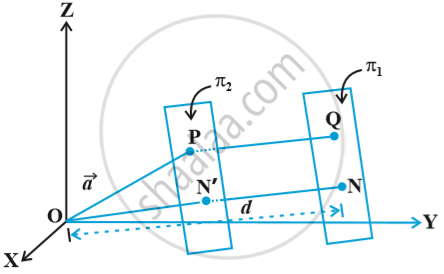# Distance of a Point from a Plane

#### notes

Consider a point P with position vector vec a and a plane π_1 whose equation is vec r . hat n = d Fig.Consider a plane π_2 through P parallel to the plane π_1. The unit vector normal to π_2 is
hat n.
Hence , its equation is (vec r - vec a) . hat n = 0
i.e.,  (vec r . hat n = vec a . hat n)
Thus, the distance ON′ of this plane from the origin is |vec a . hat n|. Therefore , the distance PQ from the plane π_1 is above fig.
i.e. ON - ON' = |d - vec a. hat n|
which is the length of the perpendicular from a point to the given plane.

Cartesian form
Let P(x_1, y_1, z_1) be the given point with position vectorvec a
and  Ax + By + Cz = D
be the Cartesian equation of the given plane. Then
vec a = x_1 hat i + y_1 hat j + z_1 hat k
vec N = A hat i + B hat j + C hat k
Hence, the perpendicular from P to the plane is
|((x_1 hat i + y_1 hat j + z_1 hat k) . (A hat i + B hat j + C hat k) - D)/ sqrt (A^2 + B^2 +C^2)|

=|(Ax_1 + By_1 + Cz_1 - D)/sqrt(A^2 + B^2 +C^2)|

If you would like to contribute notes or other learning material, please submit them using the button below.

#### Video Tutorials

We have provided more than 1 series of video tutorials for some topics to help you get a better understanding of the topic.

Series 1

Series 2

### Shaalaa.com

Three Dimensional Geometry Part 7 - The Plane [00:45:06]
S
0%